# Gradient Flow / Gravitational AllocationsPlanar gradient flow allocation.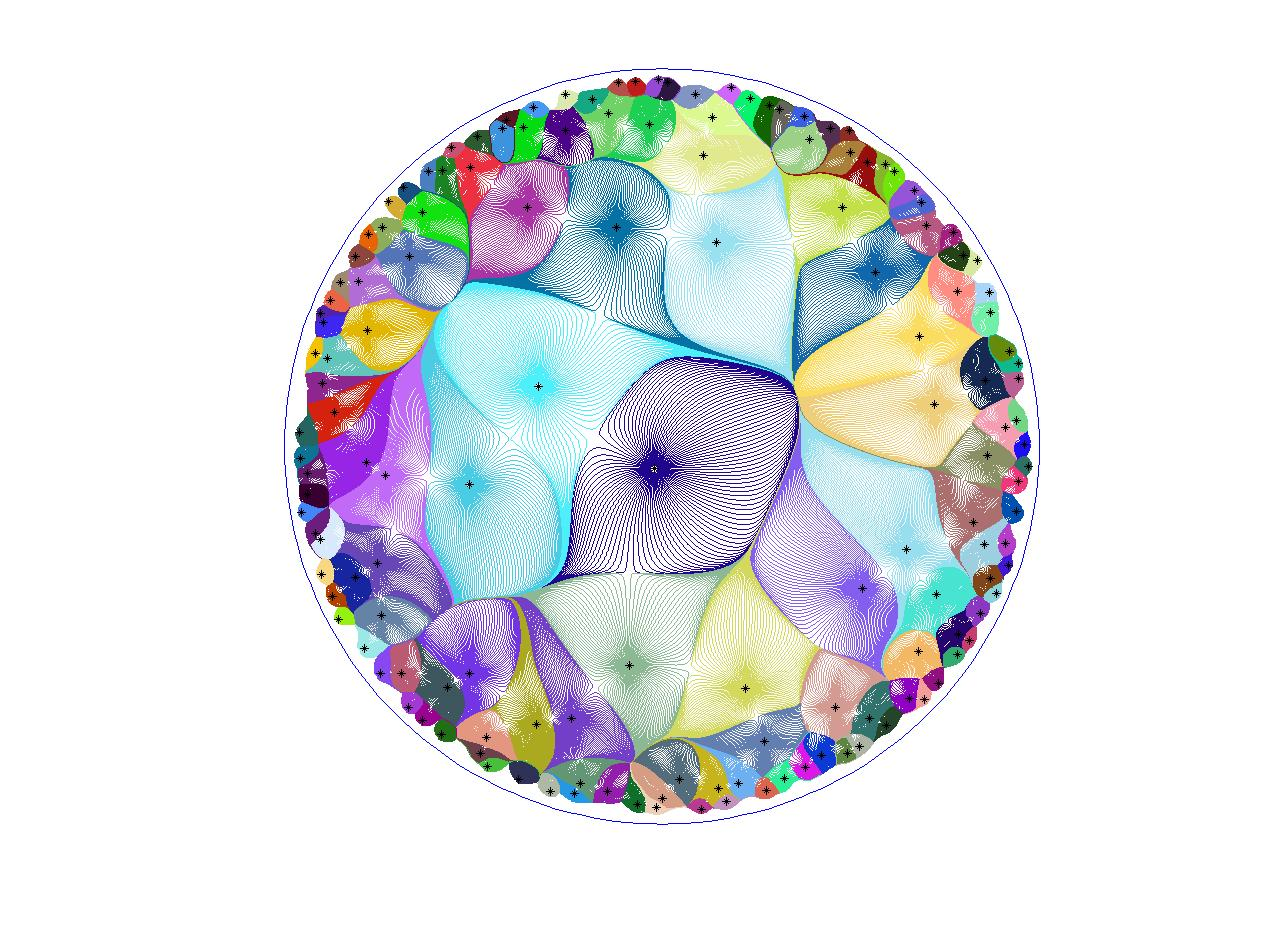Hyperbolic gradient flow allocation (10 centers per unit hyperbolic area on average).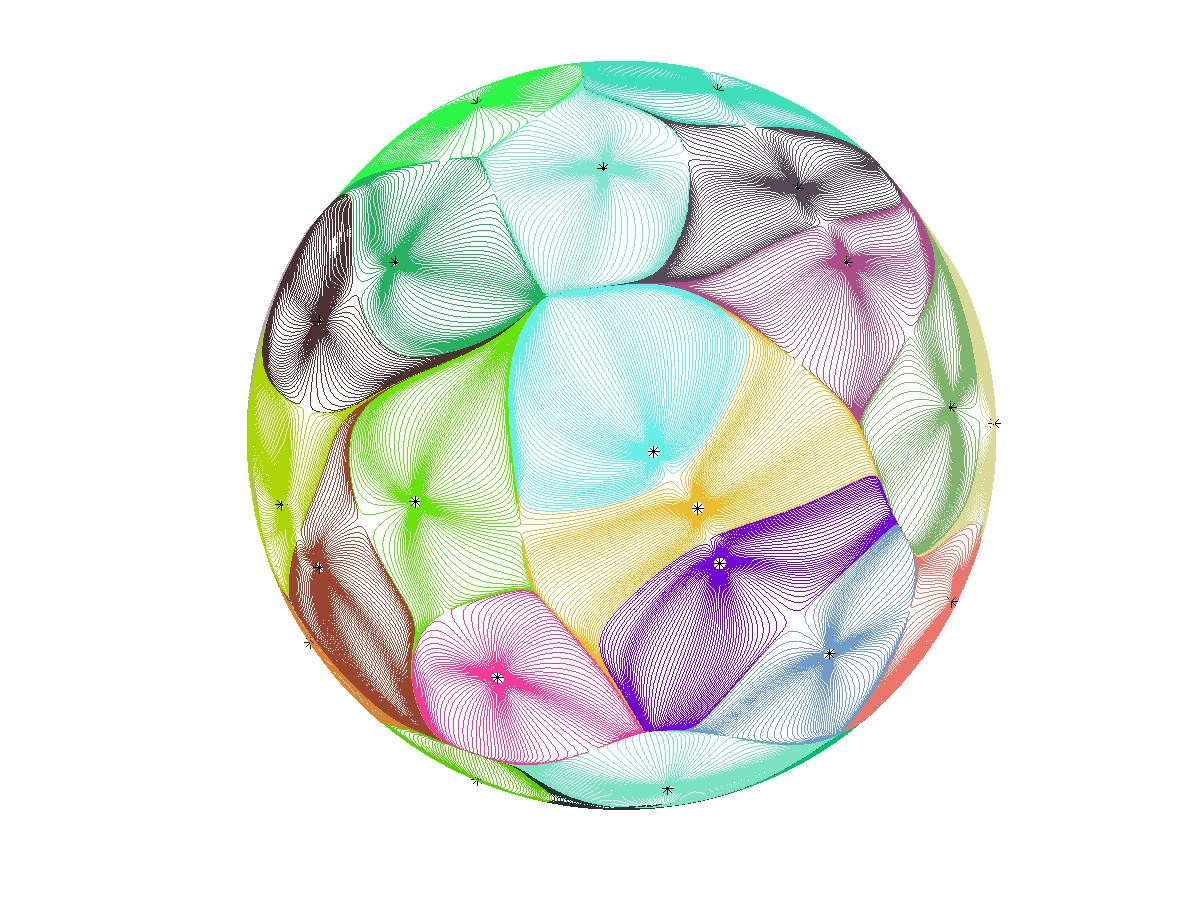Spherical gradient flow allocation (40 centers in total).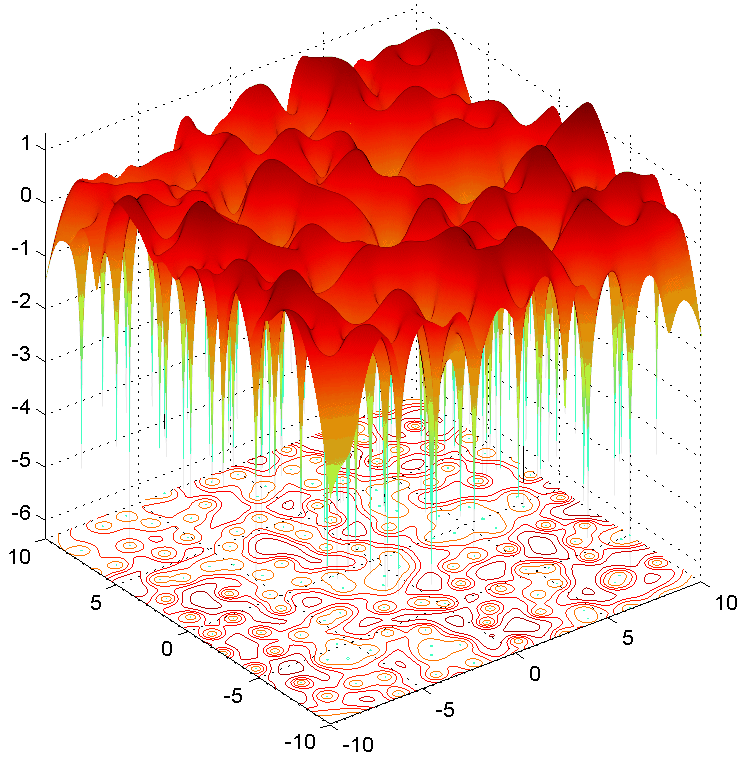Potential function for the planar gradient flow allocation.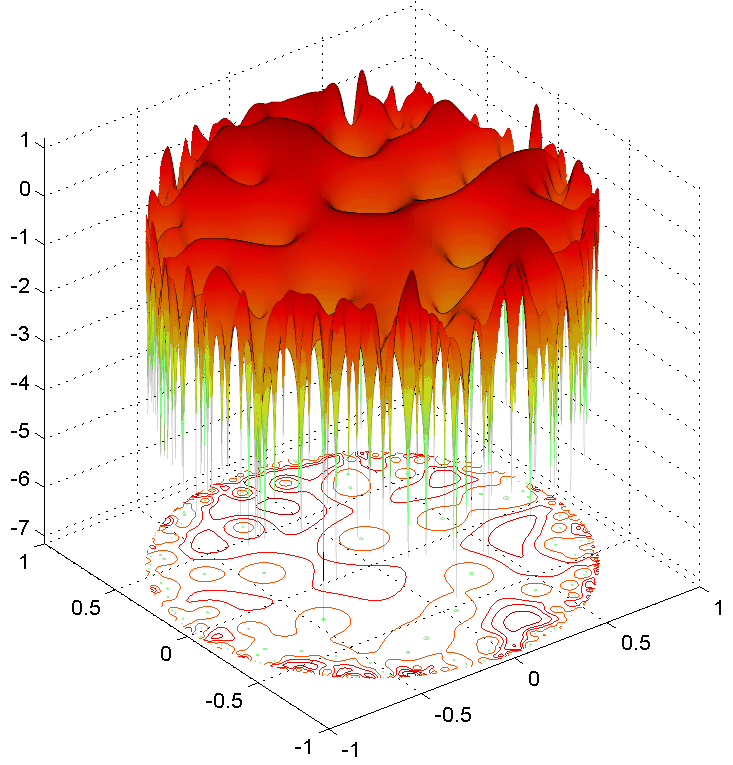Potential function for the hyperbolic gradient flow allocation.

Given a point set in the plane, an allocation is a way of partitioning the space into cells of area 1 and associating the cells with the point set. The same concept exists for point sets in space where area is replaced by volume. We are especially interested in allocations which "look the same from every point". More precisely, we are looking for allocation algorithms with the property that when applying them to the point set after first shifting it, the result is the same as first performing the allocation and then the shift. Such allocation are called (deterministic) equivariant allocations. For more on allocations in general, see Dan Romik's allocation page.

One such allocation algorithm was proposed by Sodin and Tsirelson . This algorithm is called the gradient flow allocation and a variant of it is called the Gravitational Allocation. Roughly put, the Gradient Flow / Gravitational Allocation does the following: One regards the given point set as stars. Then at each point in the plane, one puts a spaceship and observes the gravitational pull that it feels from the stars. The spaceship then proceeds to travel according to this gravitational force as if it were its velocity (unlike the usual Newtonian dynamics in which the force is the acceleration). Under this dynamics, the spaceship will eventually crash into a star. We declare that the cell of a star is all the points from which a spaceship will crash into that star. Amazingly, under such a definition all cells turn out to have equal areas!. See the slideshow from my home page for a heuristic explanation of this.

The pictures above depict the Gradient Flow Allocation. The point sets are zeros of special canonical models of Gaussian Analytic Functions (GAF's). There are three 3 such models. In one, the zeros are in the entire plane. In the second, the zeros are on the sphere. And in the third, the zeros are in the hyperbolic plane which is depicted as the unit disc. Note that in the latter case, all cells have equal hyperbolic area and hence seem smaller as they near the boundary of the disc. Sodin wrote a very nice introduction to these special GAF's  for those interested in learning more. The Gradient Flow Allocation in the entire plane was investigated in a beautiful paper of Nazarov, Sodin and Volberg . In work in preparation with Jian Ding, we explore some properties of the Gradient Flow Allocation in the hyperbolic plane.

The surface plots above are the potential functions for the Gradient Flow Allocation. I.e., the holes are the zeros to which we perform the allocation and the surface is such that if you put a ball on it and let it roll (without inertia) until it lands in a hole, its (x,y) coordinates will exactly follow the flow of the spaceship described above. In yet other words, the gradient of the potential function is the gravitational force acting on the spaceship. Surface plots are drawn for the allocation in the entire plane and in the hyperbolic disc.

While the zeros of the special Gaussian Analytic Functions are canonical objects, they are not as canonical as the Poisson process. The Poisson Process is, very roughly, a collection of uniformly distributed, independent points. It is a basic object in probability theory. In joint work with Chatterjee, Peres and Romik [4,5], we investigated the Gravitational Allocation to the Poisson process points. The Poisson process has fluctuations in its distribution, having large clumps of points or big regions with no points, with greater density than the zeros of the GAF. These fluctuations do not allow us to define the allocation in two dimensions. However, we are able to define and investigate it in 3 and higher dimensions. A drawing of a 3 dimensional allocation cell is given below.

We obtain results about the geometry of the cells, and in particular about the geometry and density of irregularly elongated cells and the alignment of stars around them. We find that two mechanisms can cause a significant amount of mass to travel a long distance before crashing into a star. The mass may be attracted to a distant dense galaxy, i.e., to a location in space with an unusually high density of stars, high enough to pull the mass from far away. Or the mass may travel through a wormhole. A wormhole is a thin tube in space which is surrounded by rings of stars along its boundary. The rings become denser and denser as we go from one side of the tube to the other and they cause mass at one end of the tube to be pulled towards its other end. Depicted below are illustrations of both phenomena.

We find that for most dimensions, one of these mechanisms is more favorable than the other. In 3 dimensions, distant dense galaxies are the dominant cause for mass to travel far, whereas in 5 dimensions and higher, wormholes are the dominant cause for such travel. Interestingly, in 4 dimensions we observe a phase transition in which the dominant cause for relatively small amounts of mass to travel far is distant dense galaxies, but the dominant cause for larger amounts of mass are wormholes.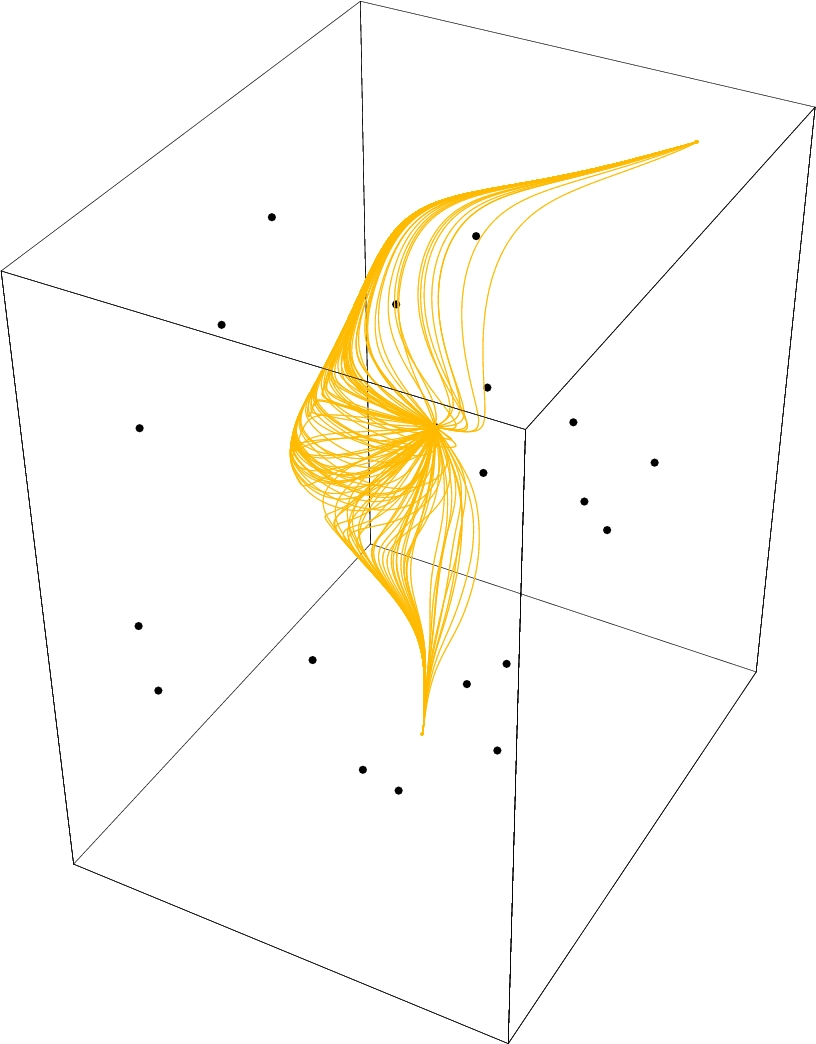A cell of the 3-dimensional gravitational allocation to Poisson points.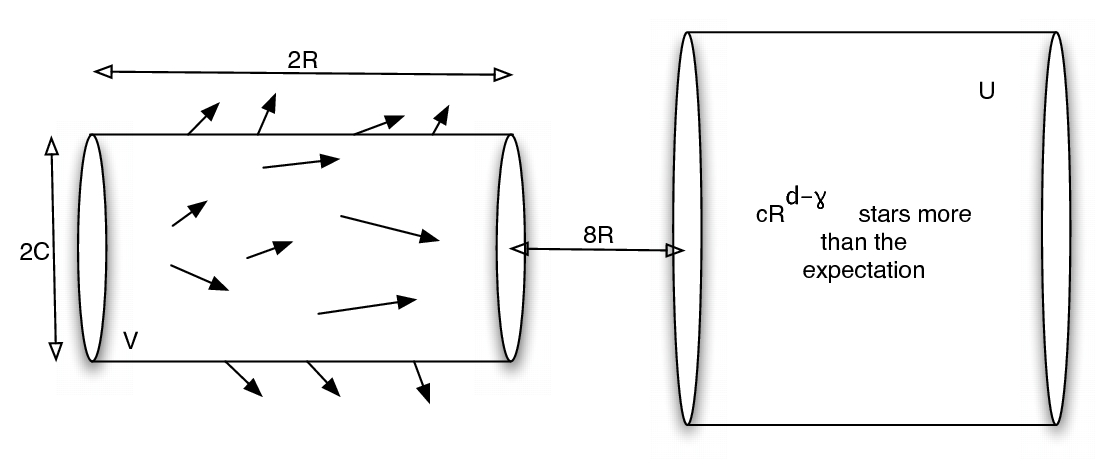An illustration of a distant dense galaxy.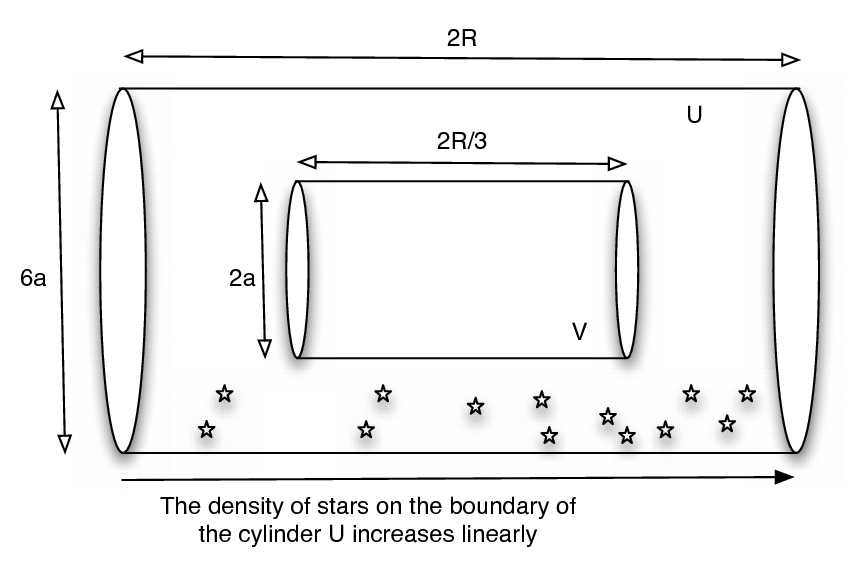An illustration of a wormhole.

### References:

 M. Sodin, B. Tsirelson. Random complex zeroes, II. Perturbed lattice. Israel Journal of Mathematics 152 (2006), 105-124.
 M. Sodin. Zeroes of Gaussian analytic functions. Talk at the 4th European Congress of Mathematics (Stockholm, 2004).
 F. Nazarov, M. Sodin and A. Volberg. Transportation to random zeroes by the gradient flow. Geometric and Functional Analysis, Vol 17-3, 887-935, 2007 (an older but still useful version 1 is also found on the arxiv).
 Chatterjee, Peled, Peres and Romik. Gravitational allocation to Poisson points. To appear in Annals of Mathematics.
 Chatterjee, Peled, Peres and Romik. Phase Transitions in Gravitational Allocation. Submitted.